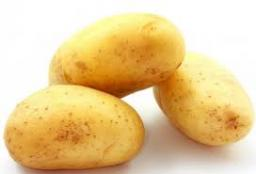# Together 4158

Daniela and Michal would dig potatoes together in 7.5 hours. But if Daniela worked alone, it would take her 2.5 hours more than Michal. Find how much Michal would do and how much Daniela would do the work himself.

d =  1.1465 h
m =  -1.3535 h

### Step-by-step explanation:Did you find an error or inaccuracy? Feel free to write us. Thank you!

Tips for related online calculators
Do you have a linear equation or system of equations and looking for its solution? Or do you have a quadratic equation?
Do you want to convert time units like minutes to seconds?